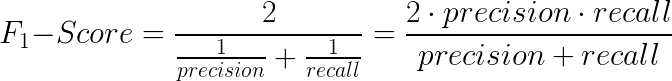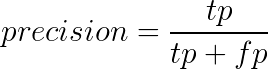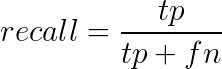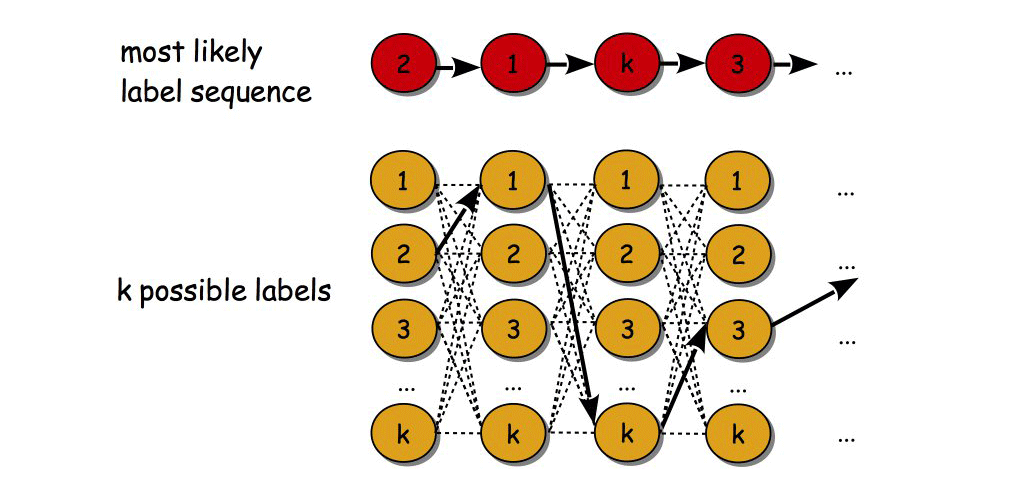# Deep Learning for information extraction: Improve your extraction results

This is the second part of a series of articles about Deep Learning methods for Natural Language Processing applications. As mentioned in the previous blog post, we will now go deeper into different strategies of extending the architecture of our system in order to improve our extraction results. This post will elaborate on techniques like word embeddings, residual connections, conditional random fields, as well as character embeddings.

## Evaluation

First, we will have a look at how we can evaluate the results of our Information Extraction system. An appropriate measure for the evaluation of an Named Entity Recognition (NER) model is the F1 score. This is defined as the harmonic mean of precision and recall:Where precision and recall are defined as:with:

tp = true positives
fp = false positives
tn = true negatives
fn = false negatives

The F1 score can be regarded as a balanced measure that puts the same weights on false negatives and false positives.

With the Python package SciKit-learn you can calculate and print these metrics for each label (see figure 1). The term support describes the number of examples found for each label in the test dataset. The average values are weighted according to the support.

## Word embeddings

Often, an improvement of the extraction results can be obtained by initializing the weights of the embedding layer with those of a pre-trained model. The idea is that the weights of the embedding are not optimized simultaneously with the rest of the neural network, but detached from it. Especially if a domain-specific text corpus is chosen, a more effective representation of the words can be achieved.

A well-known example of a statistical method for learning word vectors is the skip-gram model of word2vec . A pre-trained model can be loaded with the gensim package , as shown in listing 4.

Listing 2 shows a helper function for loading the weights of a pre-trained word vector model. This function can be used during the initialization of the network class, as listed in listing 3.

## Residual connections

With many layers, there is a certain likelihood that the intermediate representation vectors will gradually degrade. This means that the representation becomes too abstract, so that important information gets lost. On the other hand, one would like to use many layers, which can often lead to more expressive representations.

A solution to this dilemma is the use of residual connections. The original input vector is directly forwarded to all higher layers (see figure 2). The original vector is simply appended to the respective output vector of the layer. A possible implementation in PyTorch is given in listing 4.

## Conditional random fields

The decision of the hidden2tag layer in our example depends only on the input sequence. The distribution of the label sequence is not considered. A linear CRF layer implemented after the hidden2tag layer determines the probability of the entire label sequence depending on the input sequence (in this case the output sequence of the hidden2tag layer). Thus, certain patterns in the label sequence that occur very rarely or not at all in the training data can be excluded.

In figure 3, a linear CRF is represented by an undirected graph, in which only two adjacent label nodes interact with each other. This means that only the transition probabilities between two adjacent nodes are taken into account. This simplifies the calculation considerably and makes it feasible in practice.

The total probability is thus composed of the weighted product of the transition probabilities depending on the output of the hidden2tag layer in the numerator and a normalization term in the denominator (see figure 4). The weights of the counter are obtained using the maximum likelihood method. The negative log likelihood is interpreted as a loss value and replaces the cross-entropy loss function.

While the decision of the hidden2tag layer is simply obtained from the argmax() of the output, the determination of the decision sequence of the CRF layer is a bit more complicated. The goal is to find the most likely path in the label sequence (see figure 5). Due to the large number of recombinations, especially for long sequences, the brute force method is not feasible. However, decoding can be solved efficiently using dynamic programming, e.g., the Viterbi algorithm .

An implementation of the linear CRF layer including maximum likelihood and Viterbi decoding in PyTorch can be found on the Github page of AllenNLP .Figure 5: Search for the most likely path of all possible label sequences

## Character embeddings

So far, we have only considered whole words as the smallest unit. But the structure of each word can contain important information. A word can consist of a stem, prefix, and suffix. And upper and lower case can be important, too.

Well-known NLP packages like NLTK  or spaCy  provide many sophisticated linguistic tools to extract these structures and make them available as features.

Another approach is to use another neural network within the network. One option is to use a Bi-LSTM at the drawing level (see figure 6). First, each letter of a word is encoded with one-hot encoding and then read into a Bi-LSTM layer. The output of the layer can be regarded as a character-level representation of the word.

Another option is to use a character CNN (see figure 7). The individual characters are again encoded with one-hot encoding and then fitted centrally into a vector of fixed length. This vector is passed to a convolutional layer with kernel size two or three, and after a max-pooling you get an output vector, which again contains information on a character level. Listing 5 shows an example of how to implement a Bi-LSTM as character embedding.

A third option is to use fasttext  instead of word2vec. Fasttext can be seen as an extension of word2vec. In word2vec, a vector is generated for each word of a corpus, whereas fasttext generates a vector for each character-N-gram of all words in the corpus. A word, in this case, is the vector sum of all its N-grams and the word itself. Thus, not only the substructure of the words is taken into account, but also unknown words that are not in the training corpus often still get a useful vector.

## Outlook

As indicated earlier, there are promising approaches with temporal CNNs (see figure 8). Previous CNN architectures for sequence analysis have problems with long sequences. Many layers are needed to model the context dependencies of long sequences. However, this leads to the well-known problem of vanishing gradients.

A trick is now used to connect CNN layers with gaps (dilated connections). Thus, the reception field does not grow linearly, but exponentially with the number of layers, while you only need a fraction of the layers that you previously needed to extract long sequences.

CNNs have the advantage that they can be processed in parallel. Therefore they can be trained much faster than sequentially processing RNNs.

In many cases, the use of ensemble models can lead to a further improvement of the extraction results. The idea here is the combination of several, preferably diverse models (see figure 9). For example, one can take Bi-LSTM or Bi-GRU models with or without the CRF layer and those with or without character embedding. Adding a TCN model is also an option. The decision can then be composed of the majority decision or the weighted decisions of the different models.